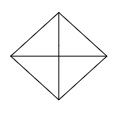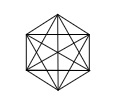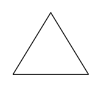# How many diagonals does each of the following have?(a) A convex quadrilateral(b) A regular hexagon(c) A triangle.

To do:

We have to find the number of diagonals each of the below shapes have.

(b) A regular hexagon
(c) A triangle.

Solution:

(a) A convex quadrilateral is shown below.From the above figure, we can observe that,

A convex quadrilateral has two diagonals.

(b) A regular hexagon is shown below.From the above figure, we can observe that,

A regular hexagon has nine diagonals.

(c) A triangle is shown below.From the above figure, we can observe that,

A triangle has no diagonals.